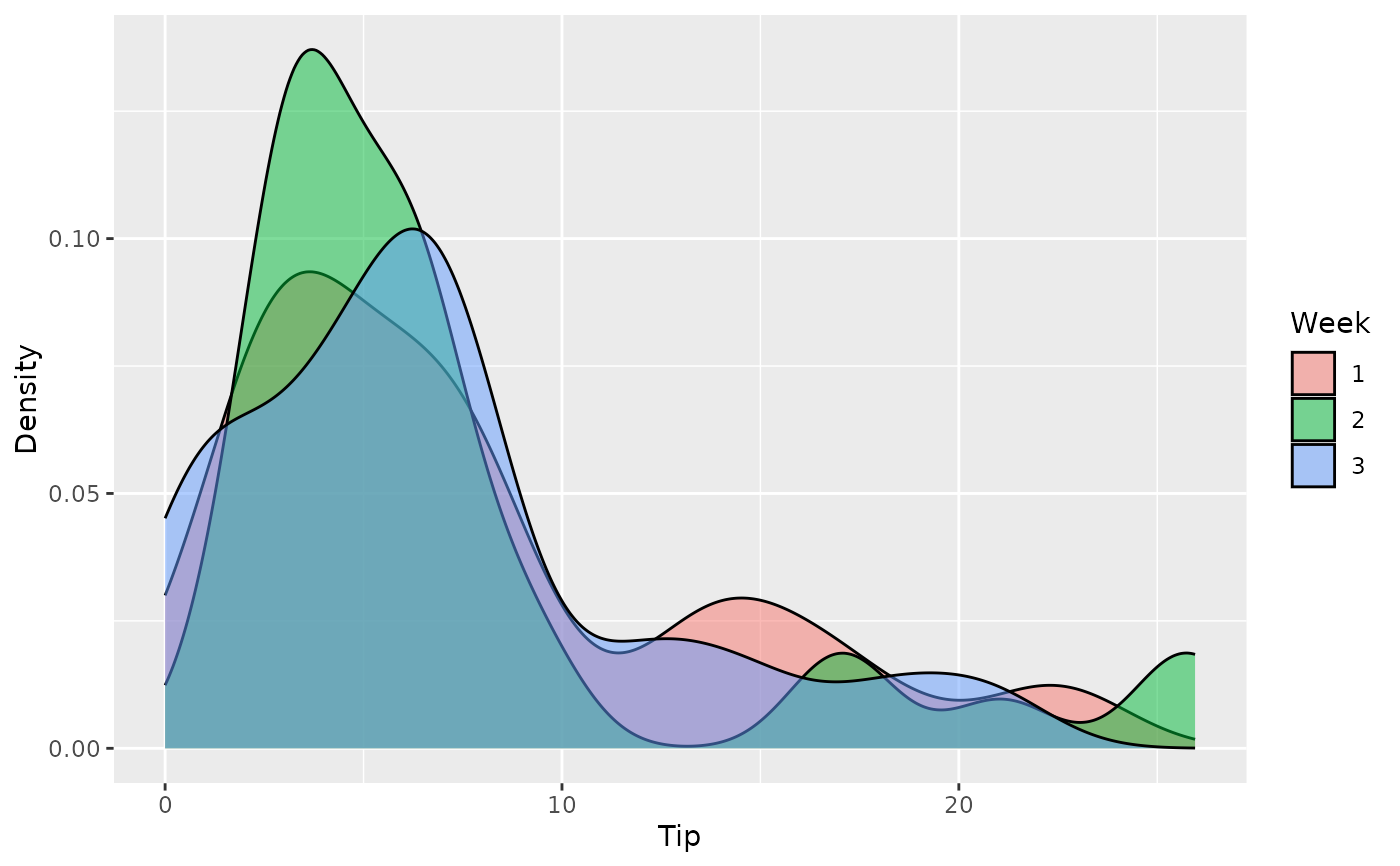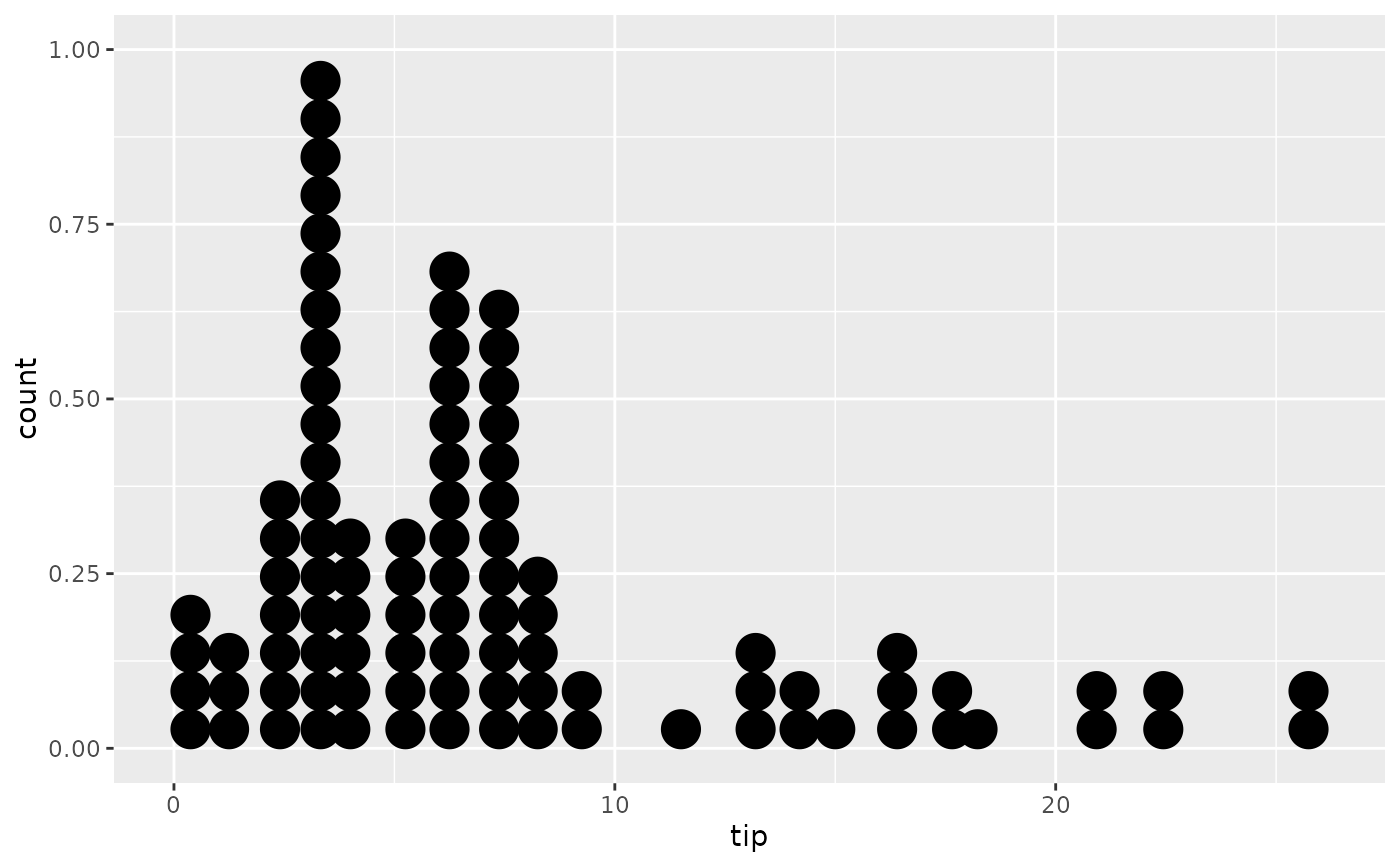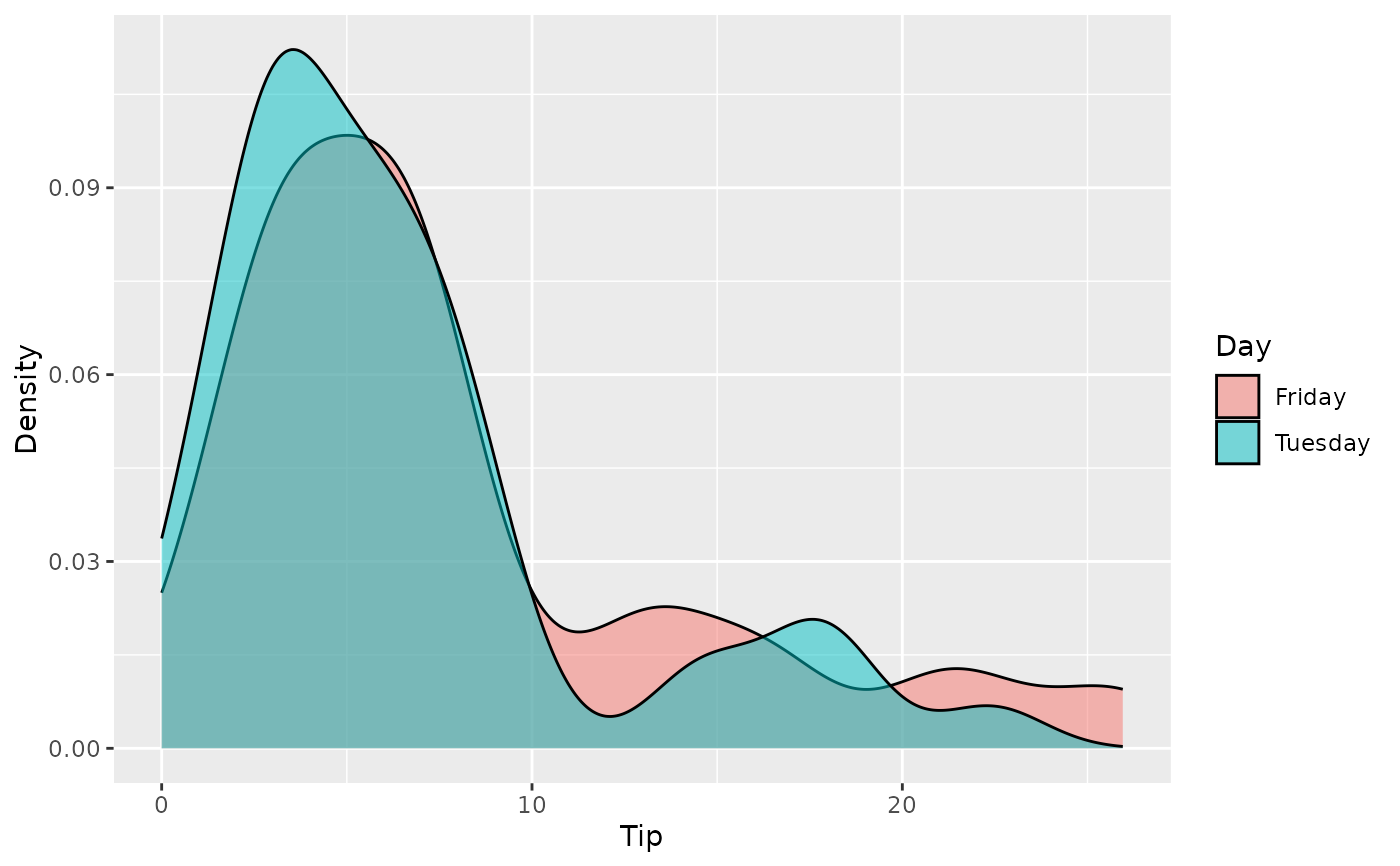A simulated data set of tips over a few weeks on a couple days per week. Each tip is associated with a single group, which may include several bills and tables (i.e. groups paid in one lump sum in simulations).

## Usage

tips

## Format

A data frame with 95 observations on the following 5 variables.

week

Week number.

day

Day, either Friday or Tuesday.

n_peop

Number of people associated with the group.

bill

Total bill for the group.

tip

Total tip from the group.

## Source

Simulated data set.

## Details

This data set was built using simulations of tables, then bills, then tips based on the bills. Large groups were assumed to only pay the gratuity, which is evident in the data. Tips were set to be plausible round values; they were often (but not always) rounded to dollars, quarters, etc.

## Examples


library(ggplot2)

ggplot(tips, aes(x = day, y = tip)) +
geom_boxplot()ggplot(tips, aes(x = tip, fill = factor(week))) +
geom_density(alpha = 0.5) +
labs(x = "Tip", y = "Density", fill = "Week")ggplot(tips, aes(x = tip)) +
geom_dotplot()
#> Bin width defaults to 1/30 of the range of the data. Pick better value with binwidth.ggplot(tips, aes(x = tip, fill = factor(day))) +
geom_density(alpha = 0.5) +
labs(x = "Tip", y = "Density", fill = "Day")